Next: 4.2.2 Boundary Models Up: 4.2 Supported Model Structures Previous: 4.2 Supported Model Structures

## 4.2.1 Volume Models

Equation (4.1) shows the structure of the partial differential equation system describing the transport of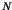quantities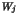within a segment of the device geometry.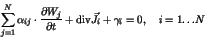(4.1)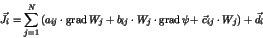(4.2)denotes the dependent variables which are the values of the affected quantities andis the number of equations.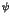is either the electrostatic potential resulting from (4.5) or can be chosen to be one of the dependent variablesby a corresponding statement in the input deck.

For a system ofimpurities the total net concentrationof all impurities is given by equation (4.3).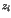denotes the charge state of the impurity(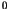for neutral impurities,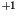for singly ionized acceptors, and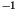for singly ionized donors).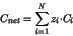(4.3)

The electrostatic potentialis determined by the Poisson equation (4.4)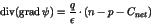(4.4)

By assuming vanishing space charge and the applicability of Boltzmann statistics the Poisson equation can be solved explicitly (4.5).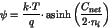(4.5)Next: 4.2.2 Boundary Models Up: 4.2 Supported Model Structures Previous: 4.2 Supported Model Structures
Robert Mlekus
1999-11-14